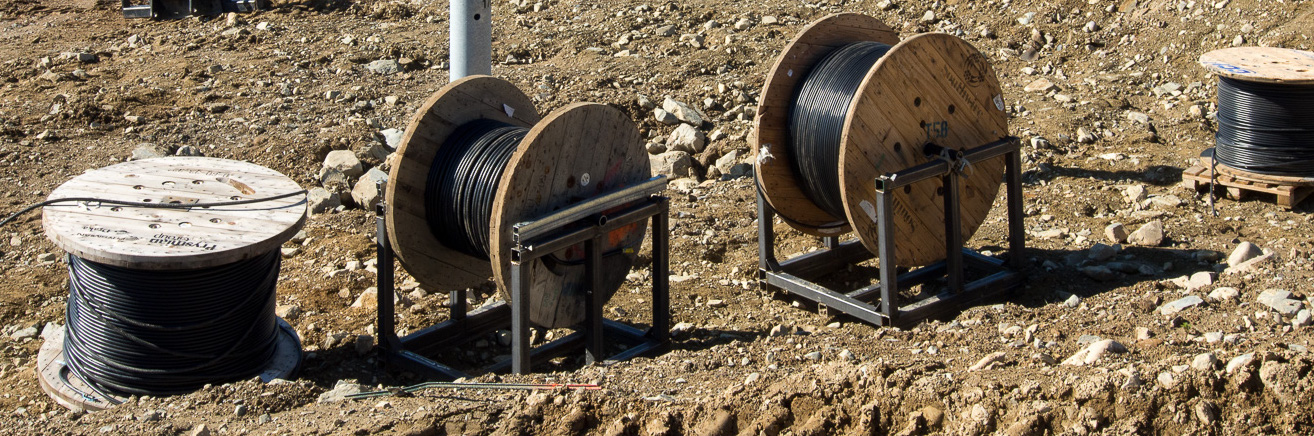# Wiring Loss

The ohmic wiring resistance induces losses both in DC – between the modules and the inverter – and AC – between the inverter and the step-up transformer.
The wire gauge (AWG) should be selected for an ohmic loss of 1.5% or less.

The formula to calculate the current are:

DC side : I = P / U or I = U / R

Where:
I: Current in Amp
U: Voltage in Volt
P: Power in Watt
R: Wire resistance in Ohm

AC side: I= P / (√3  U cos φ) or I=U / (R cos φ)

Where:
I: Current in Amp
U: Voltage in Volt (Line-to-Line)
P: Power in Watt
R: Wire resistance in Ohm
cos φ: Power factor